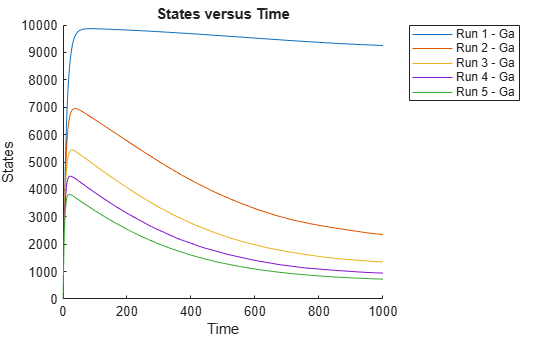Perform a Parameter Scan

This example shows how to perform a parameter scan by simulating a model multiple times, each time varying the value of a parameter.

In the model described in Model of the Yeast Heterotrimeric G Protein Cycle, the rate of G protein inactivation (kGd) is much lower in the mutant strain versus the wild-type strain (kGd = 0.004 versus kGd = 0.11), which explains higher levels of activated G protein (Ga) in the mutant strain. For a detailed look at how varying the level of kGd affects the level of Ga, perform a parameter scan over different values of kGd.

Load the gprotein.sbproj project, which includes the variable m1, a model object.

Create a vector of five evenly spaced values for kGd ranging from 0.001 to 0.15.

kGdValues = linspace(1e-3,0.15,5)';

Create a SimFunction object, where kGd is the input parameter to scan, and Ga is the observed species. Pass in an empty array [] as the last input argument to denote there are no dosed species.

simfunc = createSimFunction(m1,{'kGd'},{'Ga'},[]);

Simulate the model multiple times with different kGd values. Set the stop time to 1000.

sd = simfunc(kGdValues,1000);

Plot the simulation results to see how varying the level of kGd affects the level of Ga.

sbioplot(sd);